# Statistics review 7: Correlation and regression

## Abstract

The present review introduces methods of analyzing the relationship between two quantitative variables. The calculation and interpretation of the sample product moment correlation coefficient and the linear regression equation are discussed and illustrated. Common misuses of the techniques are considered. Tests and confidence intervals for the population parameters are described, and failures of the underlying assumptions are highlighted.

## Introduction

The most commonly used techniques for investigating the relationship between two quantitative variables are correlation and linear regression. Correlation quantifies the strength of the linear relationship between a pair of variables, whereas regression expresses the relationship in the form of an equation. For example, in patients attending an accident and emergency unit (A&E), we could use correlation and regression to determine whether there is a relationship between age and urea level, and whether the level of urea can be predicted for a given age.

## Scatter diagram

When investigating a relationship between two variables, the first step is to show the data values graphically on a scatter diagram. Consider the data given in Table 1. These are the ages (years) and the logarithmically transformed admission serum urea (natural logarithm [ln] urea) for 20 patients attending an A&E. The reason for transforming the urea levels was to obtain a more Normal distribution . The scatter diagram for ln urea and age (Fig. 1) suggests there is a positive linear relationship between these variables.

## Correlation

On a scatter diagram, the closer the points lie to a straight line, the stronger the linear relationship between two variables. To quantify the strength of the relationship, we can calculate the correlation coefficient. In algebraic notation, if we have two variables x and y, and the data take the form of n pairs (i.e. [x1, y1], [x2, y2], [x3, y3] ... [xn, yn]), then the correlation coefficient is given by the following equation: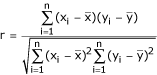whereis the mean of the x values, andis the mean of the y values.

This is the product moment correlation coefficient (or Pearson correlation coefficient). The value of r always lies between -1 and +1. A value of the correlation coefficient close to +1 indicates a strong positive linear relationship (i.e. one variable increases with the other; Fig. 2). A value close to -1 indicates a strong negative linear relationship (i.e. one variable decreases as the other increases; Fig. 3). A value close to 0 indicates no linear relationship (Fig. 4); however, there could be a nonlinear relationship between the variables (Fig. 5).

For the A&E data, the correlation coefficient is 0.62, indicating a moderate positive linear relationship between the two variables.

### Hypothesis test of correlation

We can use the correlation coefficient to test whether there is a linear relationship between the variables in the population as a whole. The null hypothesis is that the population correlation coefficient equals 0. The value of r can be compared with those given in Table 2, or alternatively exact P values can be obtained from most statistical packages. For the A&E data, r = 0.62 with a sample size of 20 is greater than the value highlighted bold in Table 2 for P = 0.01, indicating a P value of less than 0.01. Therefore, there is sufficient evidence to suggest that the true population correlation coefficient is not 0 and that there is a linear relationship between ln urea and age.

### Confidence interval for the population correlation coefficient

Although the hypothesis test indicates whether there is a linear relationship, it gives no indication of the strength of that relationship. This additional information can be obtained from a confidence interval for the population correlation coefficient.

To calculate a confidence interval, r must be transformed to give a Normal distribution making use of Fisher's z transformation :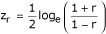The standard error  of zr is approximately: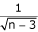and hence a 95% confidence interval for the true population value for the transformed correlation coefficient zr is given by zr - (1.96 × standard error) to zr + (1.96 × standard error). Because zr is Normally distributed, 1.96 deviations from the statistic will give a 95% confidence interval.

For the A&E data the transformed correlation coefficient zr between ln urea and age is: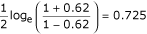The standard error of zr is: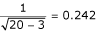The 95% confidence interval for zr is therefore 0.725 - (1.96 × 0.242) to 0.725 + (1.96 × 0.242), giving 0.251 to 1.199.

We must use the inverse of Fisher's transformation on the lower and upper limits of this confidence interval to obtain the 95% confidence interval for the correlation coefficient. The lower limit is: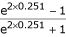giving 0.25 and the upper limit is: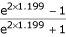giving 0.83. Therefore, we are 95% confident that the population correlation coefficient is between 0.25 and 0.83.

The width of the confidence interval clearly depends on the sample size, and therefore it is possible to calculate the sample size required for a given level of accuracy. For an example, see Bland .

### Misuse of correlation

There are a number of common situations in which the correlation coefficient can be misinterpreted.

One of the most common errors in interpreting the correlation coefficient is failure to consider that there may be a third variable related to both of the variables being investigated, which is responsible for the apparent correlation. Correlation does not imply causation. To strengthen the case for causality, consideration must be given to other possible underlying variables and to whether the relationship holds in other populations.

A nonlinear relationship may exist between two variables that would be inadequately described, or possibly even undetected, by the correlation coefficient.

A data set may sometimes comprise distinct subgroups, for example males and females. This could result in clusters of points leading to an inflated correlation coefficient (Fig. 6). A single outlier may produce the same sort of effect.

It is important that the values of one variable are not determined in advance or restricted to a certain range. This may lead to an invalid estimate of the true correlation coefficient because the subjects are not a random sample.

Another situation in which a correlation coefficient is sometimes misinterpreted is when comparing two methods of measurement. A high correlation can be incorrectly taken to mean that there is agreement between the two methods. An analysis that investigates the differences between pairs of observations, such as that formulated by Bland and Altman , is more appropriate.

## Regression

In the A&E example we are interested in the effect of age (the predictor or x variable) on ln urea (the response or y variable). We want to estimate the underlying linear relationship so that we can predict ln urea (and hence urea) for a given age. Regression can be used to find the equation of this line. This line is usually referred to as the regression line.

Note that in a scatter diagram the response variable is always plotted on the vertical (y) axis.

### Equation of a straight line

The equation of a straight line is given by y = a + bx, where the coefficients a and b are the intercept of the line on the y axis and the gradient, respectively. The equation of the regression line for the A&E data (Fig. 7) is as follows: ln urea = 0.72 + (0.017 × age) (calculated using the method of least squares, which is described below). The gradient of this line is 0.017, which indicates that for an increase of 1 year in age the expected increase in ln urea is 0.017 units (and hence the expected increase in urea is 1.02 mmol/l). The predicted ln urea of a patient aged 60 years, for example, is 0.72 + (0.017 × 60) = 1.74 units. This transforms to a urea level of e1.74 = 5.70 mmol/l. The y intercept is 0.72, meaning that if the line were projected back to age = 0, then the ln urea value would be 0.72. However, this is not a meaningful value because age = 0 is a long way outside the range of the data and therefore there is no reason to believe that the straight line would still be appropriate.

### Method of least squares

The regression line is obtained using the method of least squares. Any line y = a + bx that we draw through the points gives a predicted or fitted value of y for each value of x in the data set. For a particular value of x the vertical difference between the observed and fitted value of y is known as the deviation, or residual (Fig. 8). The method of least squares finds the values of a and b that minimise the sum of the squares of all the deviations. This gives the following formulae for calculating a and b: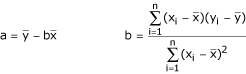Usually, these values would be calculated using a statistical package or the statistical functions on a calculator.

### Hypothesis tests and confidence intervals

We can test the null hypotheses that the population intercept and gradient are each equal to 0 using test statistics given by the estimate of the coefficient divided by its standard error.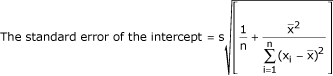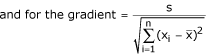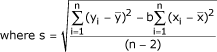The test statistics are compared with the t distribution on n - 2 (sample size - number of regression coefficients) degrees of freedom .

The 95% confidence interval for each of the population coefficients are calculated as follows: coefficient ± (tn-2 × the standard error), where tn-2 is the 5% point for a t distribution with n - 2 degrees of freedom.

For the A&E data, the output (Table 3) was obtained from a statistical package. The P value for the coefficient of ln urea (0.004) gives strong evidence against the null hypothesis, indicating that the population coefficient is not 0 and that there is a linear relationship between ln urea and age. The coefficient of ln urea is the gradient of the regression line and its hypothesis test is equivalent to the test of the population correlation coefficient discussed above. The P value for the constant of 0.054 provides insufficient evidence to indicate that the population coefficient is different from 0. Although the intercept is not significant, it is still appropriate to keep it in the equation. There are some situations in which a straight line passing through the origin is known to be appropriate for the data, and in this case a special regression analysis can be carried out that omits the constant .

### Analysis of variance

As stated above, the method of least squares minimizes the sum of squares of the deviations of the points about the regression line. Consider the small data set illustrated in Fig. 9. This figure shows that, for a particular value of x, the distance of y from the mean of y (the total deviation) is the sum of the distance of the fitted y value from the mean (the deviation explained by the regression) and the distance from y to the line (the deviation not explained by the regression).

The regression line for these data is given by y = 6 + 2x. The observed, fitted values and deviations are given in Table 4. The sum of squared deviations can be compared with the total variation in y, which is measured by the sum of squares of the deviations of y from the mean of y. Table 4 illustrates the relationship between the sums of squares. Total sum of squares = sum of squares explained by the regression line + sum of squares not explained by the regression line. The explained sum of squares is referred to as the 'regression sum of squares' and the unexplained sum of squares is referred to as the 'residual sum of squares'.

This partitioning of the total sum of squares can be presented in an analysis of variance table (Table 5). The total degrees of freedom = n - 1, the regression degrees of freedom = 1, and the residual degrees of freedom = n - 2 (total - regression degrees of freedom). The mean squares are the sums of squares divided by their degrees of freedom.

If there were no linear relationship between the variables then the regression mean squares would be approximately the same as the residual mean squares. We can test the null hypothesis that there is no linear relationship using an F test. The test statistic is calculated as the regression mean square divided by the residual mean square, and a P value may be obtained by comparison of the test statistic with the F distribution with 1 and n - 2 degrees of freedom . Usually, this analysis is carried out using a statistical package that will produce an exact P value. In fact, the F test from the analysis of variance is equivalent to the t test of the gradient for regression with only one predictor. This is not the case with more than one predictor, but this will be the subject of a future review. As discussed above, the test for gradient is also equivalent to that for the correlation, giving three tests with identical P values. Therefore, when there is only one predictor variable it does not matter which of these tests is used.

The analysis of variance for the A&E data (Table 6) gives a P value of 0.006 (the same P value as obtained previously), again indicating a linear relationship between ln urea and age.

### Coefficent of determination

Another useful quantity that can be obtained from the analysis of variance is the coefficient of determination (R2).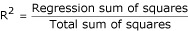It is the proportion of the total variation in y accounted for by the regression model. Values of R2 close to 1 imply that most of the variability in y is explained by the regression model. R2 is the same as r2 in regression when there is only one predictor variable.

For the A&E data, R2 = 1.462/3.804 = 0.38 (i.e. the same as 0.622), and therefore age accounts for 38% of the total variation in ln urea. This means that 62% of the variation in ln urea is not accounted for by age differences. This may be due to inherent variability in ln urea or to other unknown factors that affect the level of ln urea.

### Prediction

The fitted value of y for a given value of x is an estimate of the population mean of y for that particular value of x. As such it can be used to provide a confidence interval for the population mean . The fitted values change as x changes, and therefore the confidence intervals will also change.

The 95% confidence interval for the fitted value of y for a particular value of x, say xp, is again calculated as fitted y ± (tn-2 × the standard error). The standard error is given by: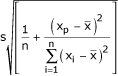Fig. 10 shows the range of confidence intervals for the A&E data. For example, the 95% confidence interval for the population mean ln urea for a patient aged 60 years is 1.56 to 1.92 units. This transforms to urea values of 4.76 to 6.82 mmol/l.

The fitted value for y also provides a predicted value for an individual, and a prediction interval or reference range  can be obtained (Fig. 10). The prediction interval is calculated in the same way as the confidence interval but the standard error is given by: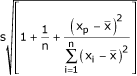For example, the 95% prediction interval for the ln urea for a patient aged 60 years is 0.97 to 2.52 units. This transforms to urea values of 2.64 to 12.43 mmol/l.

Both confidence intervals and prediction intervals become wider for values of the predictor variable further from the mean.

## Assumptions and limitations

The use of correlation and regression depends on some underlying assumptions. The observations are assumed to be independent. For correlation both variables should be random variables, but for regression only the response variable y must be random. In carrying out hypothesis tests or calculating confidence intervals for the regression parameters, the response variable should have a Normal distribution and the variability of y should be the same for each value of the predictor variable. The same assumptions are needed in testing the null hypothesis that the correlation is 0, but in order to interpret confidence intervals for the correlation coefficient both variables must be Normally distributed. Both correlation and regression assume that the relationship between the two variables is linear.

A scatter diagram of the data provides an initial check of the assumptions for regression. The assumptions can be assessed in more detail by looking at plots of the residuals [4, 7]. Commonly, the residuals are plotted against the fitted values. If the relationship is linear and the variability constant, then the residuals should be evenly scattered around 0 along the range of fitted values (Fig. 11).

In addition, a Normal plot of residuals can be produced. This is a plot of the residuals against the values they would be expected to take if they came from a standard Normal distribution (Normal scores). If the residuals are Normally distributed, then this plot will show a straight line. (A standard Normal distribution is a Normal distribution with mean = 0 and standard deviation = 1.) Normal plots are usually available in statistical packages.

Figs 12 and 13 show the residual plots for the A&E data. The plot of fitted values against residuals suggests that the assumptions of linearity and constant variance are satisfied. The Normal plot suggests that the distribution of the residuals is Normal.

When using a regression equation for prediction, errors in prediction may not be just random but also be due to inadequacies in the model. In particular, extrapolating beyond the range of the data is very risky.

A phenomenon to be aware of that may arise with repeated measurements on individuals is regression to the mean. For example, if repeat measures of blood pressure are taken, then patients with higher than average values on their first reading will tend to have lower readings on their second measurement. Therefore, the difference between their second and first measurements will tend to be negative. The converse is true for patients with lower than average readings on their first measurement, resulting in an apparent rise in blood pressure. This could lead to misleading interpretations, for example that there may be an apparent negative correlation between change in blood pressure and initial blood pressure.

## Conclusion

Both correlation and simple linear regression can be used to examine the presence of a linear relationship between two variables providing certain assumptions about the data are satisfied. The results of the analysis, however, need to be interpreted with care, particularly when looking for a causal relationship or when using the regression equation for prediction. Multiple and logistic regression will be the subject of future reviews.

## Abbreviations

AE:

accident and emergency unit

ln:

natural logarithm (logarithm base e).

## References

1. Whitley E, Ball J: Statistics review 1: Presenting and summarising data. Crit Care 2002, 6: 66-71. 10.1186/cc1455

2. Kirkwood BR, Sterne JAC: Essential Medical Statistics 2 Edition Oxford: Blackwell Science 2003.

3. Whitley E, Ball J: Statistics review 2: Samples and populations. Crit Care 2002, 6: 143-148. 10.1186/cc1473

4. Bland M: An Introduction to Medical Statistics 3 Edition Oxford: Oxford University Press 2001.

5. Bland M, Altman DG: Statistical methods for assessing agreement between two methods of clinical measurement. Lancet 1986, i: 307-310.

6. Zar JH: Biostatistical Analysis 4 Edition New Jersey, USA: Prentice Hall 1999.

7. Altman DG: Practical Statistics for Medical Research London: Chapman & Hall 1991.

## Author information

Authors

### Corresponding author

Correspondence to Viv Bewick.

None declared.

## Rights and permissions

Reprints and Permissions

Bewick, V., Cheek, L. & Ball, J. Statistics review 7: Correlation and regression. Crit Care 7, 451 (2003). https://doi.org/10.1186/cc2401